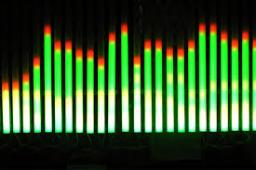# Hundred 2808

Three times the unknown number minus ten is less than one hundred, as one hundred is more than twice that number. Which number is it?

x =  42

### Step-by-step explanation:Did you find an error or inaccuracy? Feel free to write us. Thank you!

Tips for related online calculators
Do you have a linear equation or system of equations and looking for its solution? Or do you have a quadratic equation?